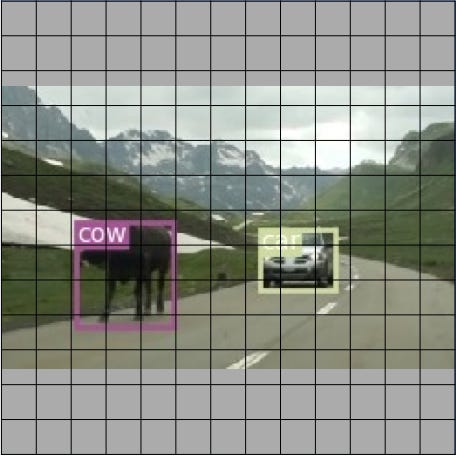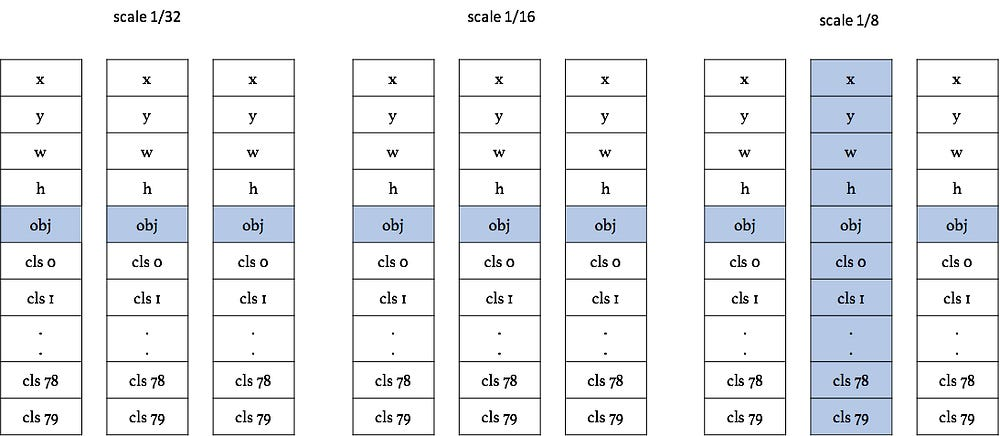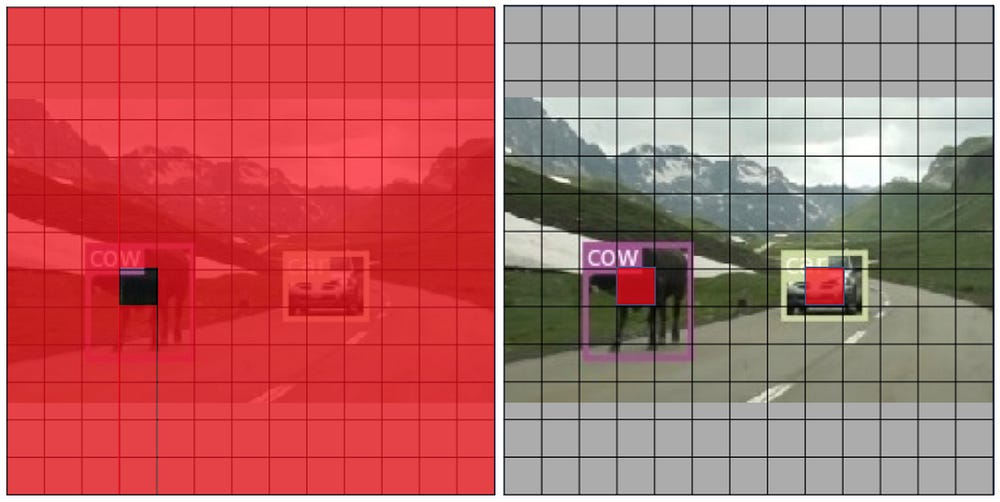# Reproducing training performance of YOLOv3 in PyTorch (Part2)

Source: Deep Learning on Medium

### Part 2: How to assign targets to multi-scale anchors

Hi, I’m Hiroto Honda, an R&D engineer at DeNA Co., Ltd. in Japan.

In this article, I will share the details for training the YOLOv3 detector, which are implemented in our PyTorch_YOLOv3 repository that was open-sourced by DeNA on Dec. 6, 2018.
Last time I introduced the details of the network architecture and the roles of the channels in the detection (yolo) layers. This time, I would like to explain how the ground truth data are assigned to the target tensor, which is the core idea of the detector. Just as in the previous post, the details in this post are not written in the paper, but in the original implementation.

### Contents

1. Target tensor and anchors
2. Target assignment using IoU calculation
3. xywh channels
4. class channels
5. objectness channels

Let’s see how the ground truth (GT) boxes are assigned as a target tensor using the example shown in Fig. 1.Fig. 1 Mountain pass example again. The image is gridded into 13 x 13.

### 1. Target tensor and anchors

To train the YOLO detector, we have to convert the annotation data to the ‘target tensor’. The target tensor has exactly the same dimensions as the tensor in the yolo layers. Let’s see the channel map introduced in the previous story (Fig. 2):

### 2. Target assignment using IoU calculation

To pick an anchor that is the most similar to each ground truth (GT) box, we calculate intersection over Union (IoU) between each GT box and anchors across three scales, and assign the anchor with the highest IoU value. Note that every GT box is assigned to an anchor, but not every anchor is not assigned to by a GT box.

For example, the GT box of the car in Fig. 1 has the highest IoU with the second anchor in the 1/8 scale. The channels of the target tensor used for loss calculation are highlighted in Fig. 3. Let’s see what this means in the following sections.Fig. 3 Target assignment on all the channels at the ‘car’ grid.

### 3. xywh channels

Now a GT target is assigned to an anchor.

As I introduced in Part 1, the target of the x, y channels are the relative positions within the grid box : (σ(x), σ(y)), where σ is a sigmoid function whose output values range from 0 to 1. For w channels, the target channel values are set to log(w_GT / w_a ) using the anchor width w_a and the ground truth box width w_GT. The same goes for h channels.

Note that non-assigned target channels are ‘ignored’ and masked when calculating the loss.

### 4. class channels

As introduced in Part 1, there are 80 class channels per each anchor (for COCO dataset). If the class id of the GT label is 67, the 67th class channel of the target tensor is set to one and the others to zero. As well as the xywh channels, non-assigned target channels are masked for loss calculation.

### 5. objectness channels

Target assignment for the obj channel is different from the others (see Fig. 4). We have to learn objectness at ‘background’ grids to ensure the detector can tell there are no objects in the grids.

Background grid:

Obj channels should be close to zero when no objects are in the grid, so all the obj channels’ targets except for the assigned anchor are set to zero (not ignored!).

Foreground grid:

As for the assigned anchor channel, the target is set to one.

When IoU between the current prediction and the GT box is more than the predefined threshold (0.7 in default), the channel is ignored when calculating the loss (just like non-assigned xywh and class channels).Fig. 4 Target assignment for objectness (left) and the other channels (right). Only the channels at highlighted grids are used for loss calculation and the rest is ignored. Note that the objectness at the center of ‘cow’ is to be ignored due to high IoU between the prediction and ground truth boxes.

Finally we have set all the channels of the target tensor. Now we are able to compare the target and prediction tensors to calculate loss.

That’s it for this time. Next time, I am going to explain the actual loss functions used to train the detector.

Part 1. Network Architecture and channel elements of YOLO layers

Part 2. How to assign targets to multi-scale anchors

Part 3. What are the actual loss functions?

Check out our PyTorch implementation of YOLOv3!!

https://github.com/DeNA/PyTorch_YOLOv3

Thank you, see you again in Part 3!